시간 제한메모리 제한제출정답맞은 사람정답 비율
8 초 256 MB0000.000%

## 문제

Texas hold 'em (also known as Texas holdem, hold 'em, and holdem) is one of the most popular variants of the card game of poker. Please read the following rules as they may be different from the regular rules.

Now, two players, Alice and Bob, are playing Texas holdem. At the beginning of each game, each player keeps two cards, known as hole cards. In this problem, different from the regular Texas holdem, where the hole cards are put face-down, both players know all the four hole cards. Then, five community cards are dealt in three stages face-up. The stages consist of a series of three cards ("the flop"), later an additional single card ("the turn" or "fourth street"), and a final card ("the river" or "fifth street"). All players know the face-up cards that are already dealt.

All cards are drawn from a standard 52-card deck. A standard 52-card deck comprises 13 ranks in each of the four French suits: clubs ($\clubsuit$), diamonds ($\diamondsuit$), hearts ($\heartsuit$), and spades ($\spadesuit$). Each suit includes an Ace (A), a King (K), Queen (Q) and Jack (J), each depicted alongside a symbol of its suit; and numerals or pip cards from the Deuce (Two) to the Ten, with each card depicting that many symbols (pips) of its suit. No card can be drawn more than once.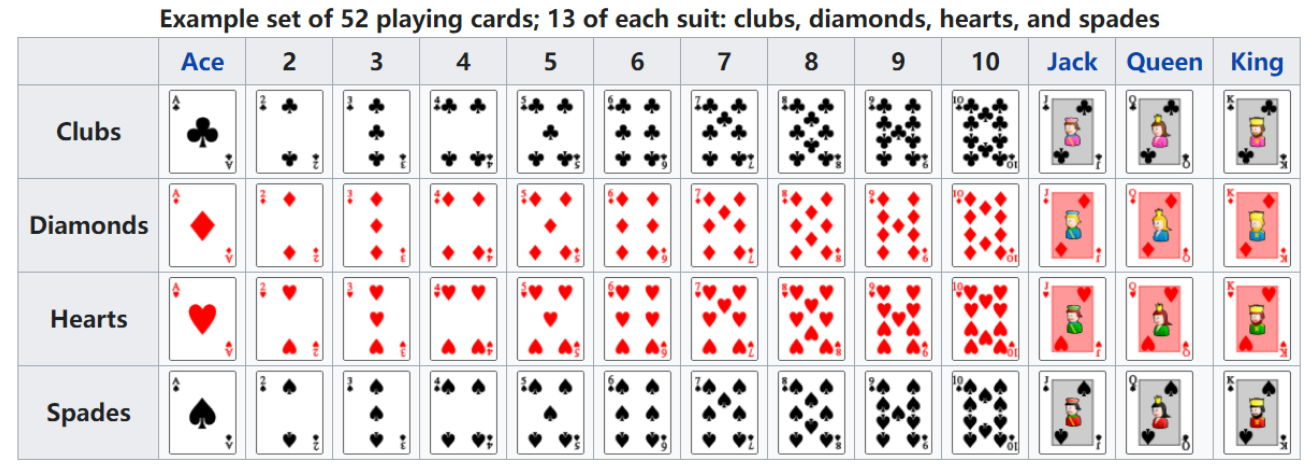Individual cards are ranked as follows (high-to-low): Ace, King, Queen, Jack, 10, 9, 8, 7, 6, 5, 4, 3, 2. Each player seeks the best five-card poker hand from any combination of the seven cards --- the five community cards and the player's two hole cards.

The following table shows the possible five-card poker hand types in increasing order of their values. Each type has a specific ordering of the five cards that are described below. The following part is describing how to compare two hands, which is the same as the regular rule.

Highcard: Simple value of the card. The cards are ordered as $a_1 a_2 a_3 a_4 a_5$ such that $a_1 > a_2 > a_3 > a_4 > a_5$.

Here and below, $a_i$ represents the rank of $i$-th card. Note that, while the pictures here and below show examples of hand types, the cards on the pictures may not yet be ordered properly.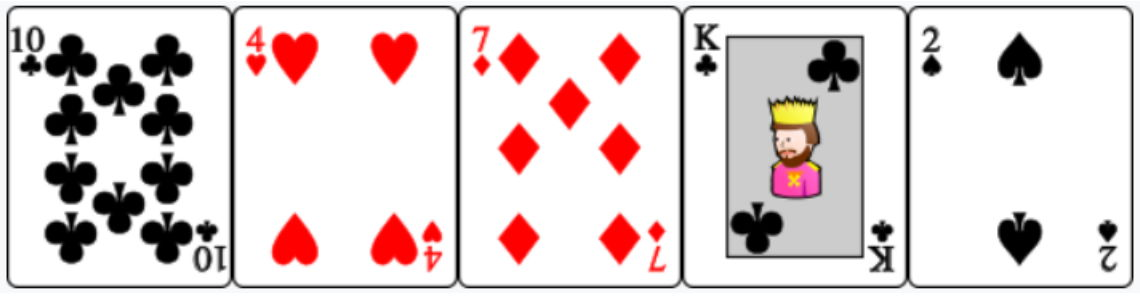Pair: Two cards with the same value. The cards are ordered as $a_1 a_2 a_3 a_4 a_5$ such that $a_1 = a_2$, $a_3 > a_4 > a_5$, $a_1 \neq a_3$, $a_1 \neq a_4$, and $a_1 \neq a_5$.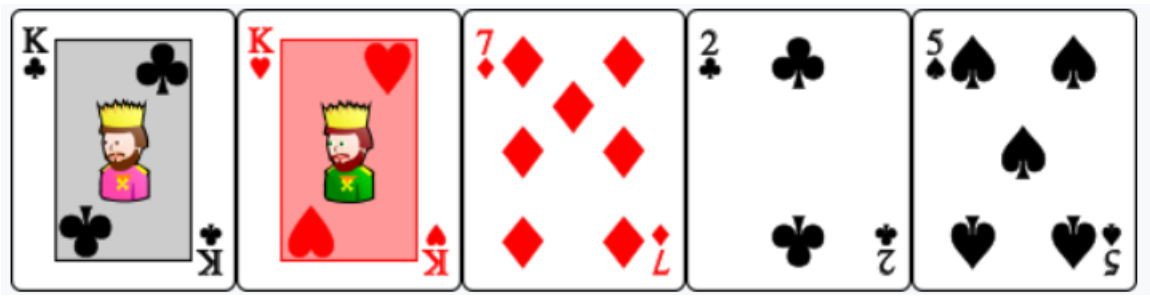Two pairs: Two times two cards with the same value. The cards are ordered as $a_1 a_2 a_3 a_4 a_5$ such that $a_1 = a_2$, $a_3 = a_4$, $a_1 > a_3$, $a_1 \neq a_5$, and $a_3 \neq a_5$.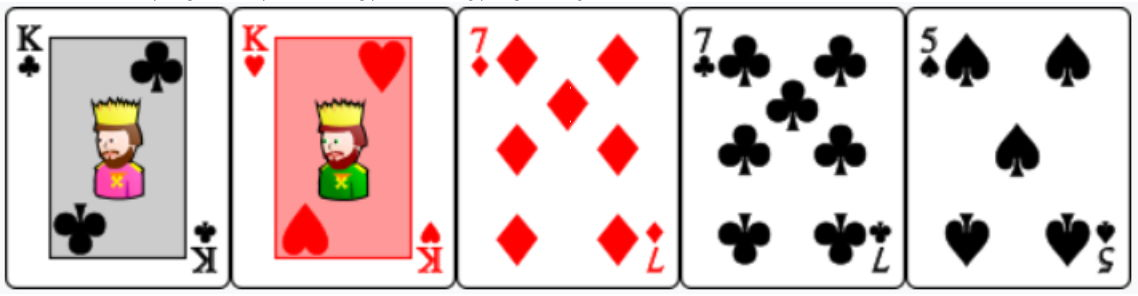Three of a kind: Three cards with the same value. The cards are ordered as $a_1 a_2 a_3 a_4 a_5$ such that $a_1 = a_2 = a_3$, $a_4 > a_5$, $a_1 \neq a_4$, and $a_1 \neq a_5$.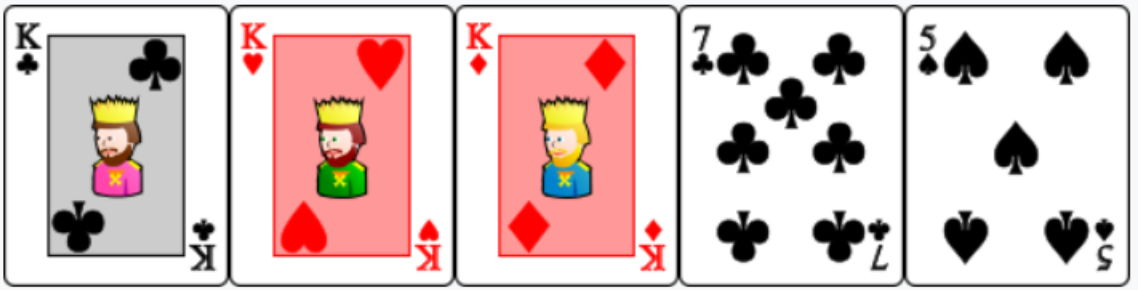Straight: Sequence of five cards in increasing value. The cards are ordered as $a_1 a_2 a_3 a_4 a_5$ such that $a_i$ is exactly one rank above $a_{i+1}$ for all $1 \leq i \leq 4$. As a special case, if $a_5$ is Ace, $a_4$ can be 2. In this case, Ace is considered one rank below 2.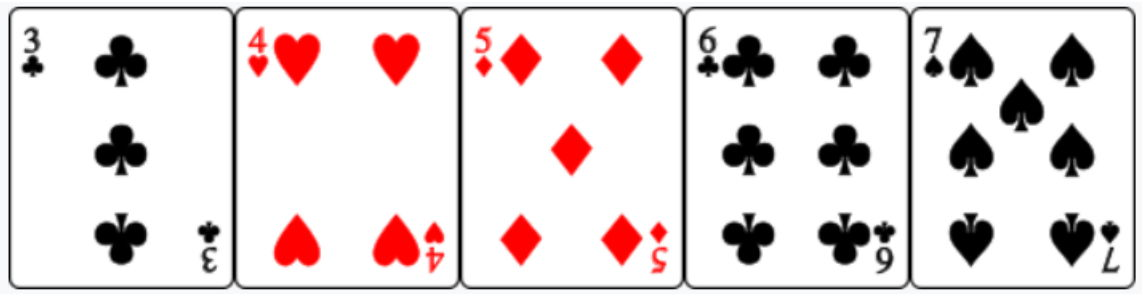Flush: Five cards of the same suit. The cards are ordered as $a_1 a_2 a_3 a_4 a_5$ such that all the five cards have the same suit and $a_1 > a_2 > a_3 > a_4 > a_5$.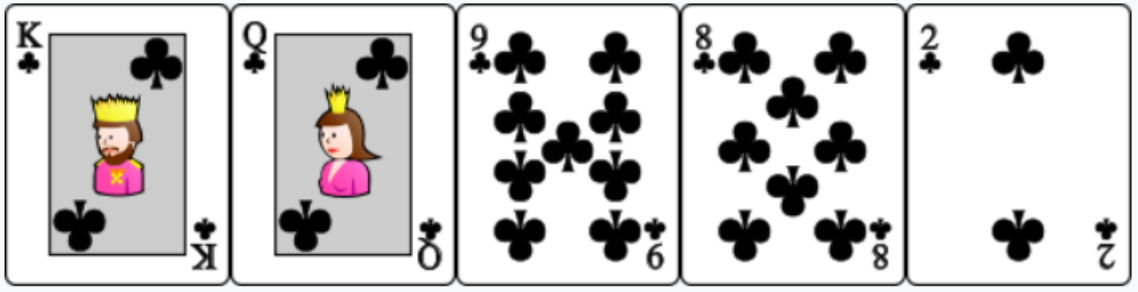Full house: Combination of three of a kind and a pair. The cards are ordered as $a_1 a_2 a_3 a_4 a_5$ such that $a_1 = a_2 = a_3$ and $a_4 = a_5$.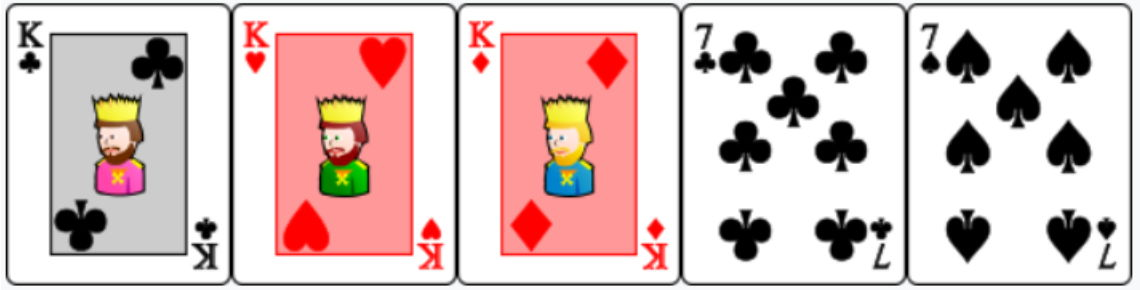Four of a kind: Four cards of the same value. The cards are ordered as $a_1 a_2 a_3 a_4 a_5$ such that $a_1 = a_2 = a_3 = a_4$.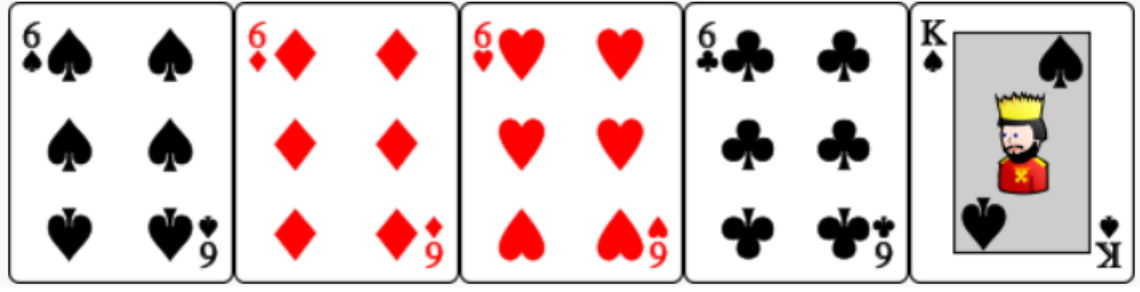Straight flush: Straight of the same suit. The cards are ordered as $a_1 a_2 a_3 a_4 a_5$ such that all the five cards have the same suit and that $a_i$ is exactly one rank above $a_{i+1}$ for all $1 \leq i \leq 4$. As a special case, if $a_5$ is Ace, $a_4$ can be 2. In this case, Ace is considered one rank below 2.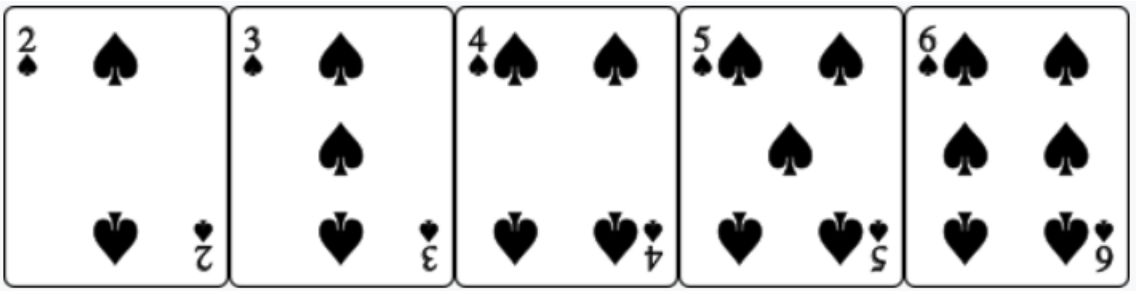To compare two hands, first, we will compare the type of two hands. For example, when one hand is Four of a kind, and the other hand is Full house, Four of a kind always wins against Full house.

If the types of two hands are the same, we compare the ranks of the cards. We will order the cards as described above, and compare them one by one. Firstly, we will compare the first card. If a hand's first card has a higher rank, it wins. If the first cards of the two hands have the same rank, we will compare the second card, and so on. If the cards have the same rank in every position, no one wins. The suit of cards never matters in this comparison. For example, $\clubsuit 5, \diamondsuit 5, \heartsuit 5, \spadesuit 2, \clubsuit 2$ wins against $\diamondsuit 3, \spadesuit 3, \heartsuit 3, \diamondsuit A, \heartsuit A$: since they are both Full house, we will first compare the ranks of the three cards of a kind, and find that $5 > 3$.

Consider the case that the hole cards of Alice are $\clubsuit A, \diamondsuit 4$ and the hole cards of Bob are $\heartsuit 2, \spadesuit 3$. The community cards are $\spadesuit A, \heartsuit 4, \spadesuit 5, \clubsuit Q, \heartsuit Q$. The best hand for Alice (five cards among her hole cards and the community cards) is $\clubsuit A, \spadesuit A, \clubsuit Q, \heartsuit Q, \spadesuit 5$, which is Two pairs. The best hand for Bob is $\spadesuit 5, \heartsuit 4, \spadesuit 3, \heartsuit 2, \spadesuit A$, which is a Straight. Thus, Bob wins.

Players have many turns to make decisions. In each turn, the player has four choices: check, call, raise, or fold. These choices will be explained later.

The game between Alice and Bob proceeds in the following way:

1. Stage 1: The $52$ cards are shuffled randomly.
• The first two cards are put face-up, as Alice's hole cards.
• The third and fourth cards are put face-up, as Bob's hole cards.
• The fifth, sixth, and seventh cards are put face-down, as the flop.
• The eighth card is put face-down, as the turn.
• The ninth card is put face-down, as the river.
Then, both Alice and Bob bet $w$ dollars, and both have $m$ dollars in hand.
2. Stage 2: A bet turn starts, where Alice and Bob bet in turns.
3. Stage 3: The flop is put face-up, then a bet turn starts.
4. Stage 4: The turn is put face-up, then a bet turn starts.
5. Stage 5: The river is put face-up, then a bet turn starts.
6. If no player chooses to fold in the above stages, their hands will be compared. Whoever has a better hand will be the winner. If the two hands are equal, the game will end with a draw.

When the game ends, suppose Alice bet $a$ dollars and Bob bet $b$ dollars. If the game ends with a draw, both players will receive $\frac{a + b}{2}$ dollars. If there is a winner, the winner will receive $a + b$ dollars and the loser will receive nothing.

If a player kept $x$ dollars in hand at the end of the game, and received $y$ dollars as the result of the game, then he or she will have a total of $x + y$ dollars when the game ends.

Each bet turn proceeds in the following way:

1. Alice's turn: Alice makes a decision.
2. Bob's turn: Bob makes a decision.
3. If Bob chooses to raise, then go back to Alice's turn. Otherwise, the bet turn ends.

Each player has four possible decisions. Suppose the current player bets $a$ dollars and the other player bets $b$ dollars. (One can prove that $b$ is always no smaller than $a$.) Then:

• check. This choice is available only when $a = b$. If a player chooses to check, nothing will happen.
• call. This choice is available only when $a < b$. Suppose the current player has $c$ dollars in the hand. When a player chooses to call, he or she has to further bet $b - a$ dollars.
• raise. This choice is always available. Suppose the current player has $c$ dollars in the hand. Then, he or she can choose an integer $d \in (b, a + c]$, and further bet $d - a$ dollars.
• fold. This choice is always available. However, when a player chooses to fold, he or she will lose the game, and the game will end immediately.

Now, Stage 1 is finished: the four hole cards are put face-up, both players have bet $w$ dollars, and both players have $m$ dollars in hand. Suppose both players play optimally, wishing to maximize the expected amount of money they have when the game ends. Your task is to calculate this maximal expected amount of money for Alice.

## 입력

The first line contains an integer $T (1 \leq T \leq 10)$, the number of test cases.

For each test case, the first line contains two integers $w$ and $m$ ($0 \leq w, m \leq 50$).

Then four lines follow. Each line contains two characters $c$ and $k$ separated by a space ($c \in \{0, 1, 2, 3\}$, $k \in \{2, 3, 4, 5, 6, 7, 8, 9, T, J, Q, K, A\})$, where $c=0, 1, 2, 3$ represent clubs ($\clubsuit$), diamonds ($\diamondsuit$), hearts ($\heartsuit$), and spades ($\spadesuit$), respectively, $k = T$ represents the card $10$, and the other values of $k$ represent the respective card values.

The first two cards represent the hole cards of Alice, and the last two cards represent the hole cards of Bob. It is guaranteed that these four cards are pairwise distinct.

## 출력

Output a single line with a single irreducible fraction: the answer to the problem. For example, when the answer is $3.5$, $0$, $1$, or $0.999$, you need to output 7/2, 0/1, 1/1, or 999/1000, respectively.

## 예제 입력 1

2
10 10
0 A
1 A
2 A
3 A
10 10
0 A
1 Q
2 K
2 J


## 예제 출력 1

20/1
557855/23782